Скачать презентацию FOL Formal Logic The most widely used

4910f187a4389061bf84818ef4011e23.ppt

• Количество слайдов: 40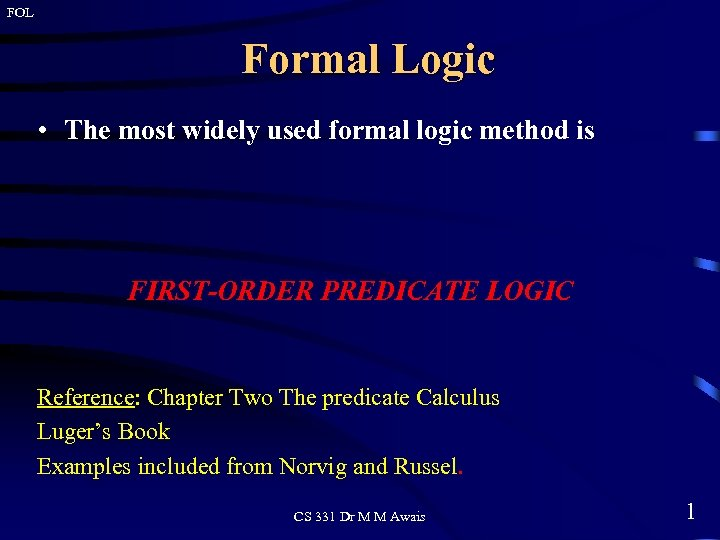FOL Formal Logic • The most widely used formal logic method is FIRST-ORDER PREDICATE LOGIC Reference: Chapter Two The predicate Calculus Luger’s Book Examples included from Norvig and Russel. CS 331 Dr M M Awais 1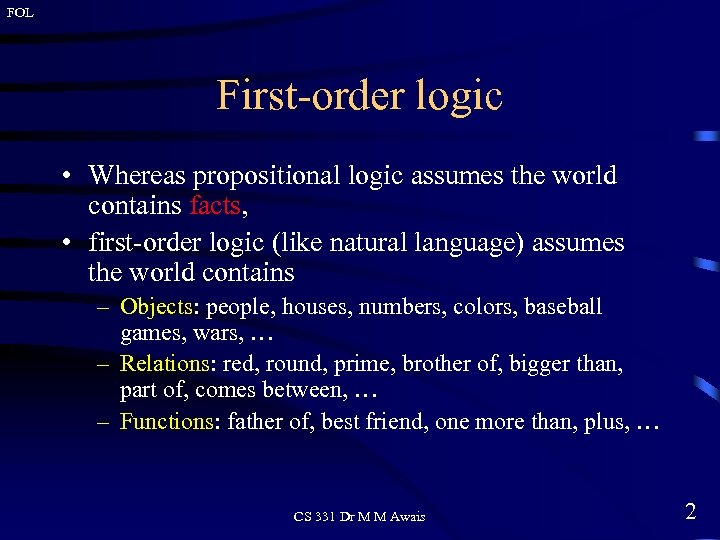FOL First-order logic • Whereas propositional logic assumes the world contains facts, • first-order logic (like natural language) assumes the world contains – Objects: people, houses, numbers, colors, baseball games, wars, … – Relations: red, round, prime, brother of, bigger than, part of, comes between, … – Functions: father of, best friend, one more than, plus, … CS 331 Dr M M Awais 2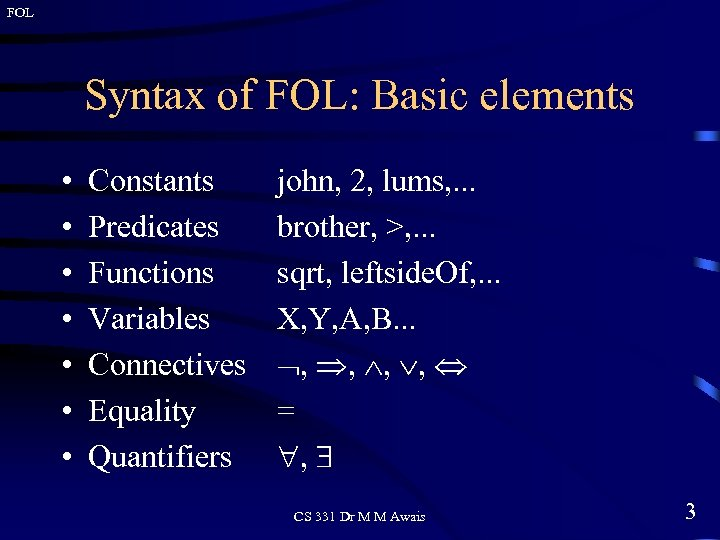FOL Syntax of FOL: Basic elements • • Constants Predicates Functions Variables Connectives Equality Quantifiers john, 2, lums, . . . brother, >, . . . sqrt, leftside. Of, . . . X, Y, A, B. . . , , = , CS 331 Dr M M Awais 3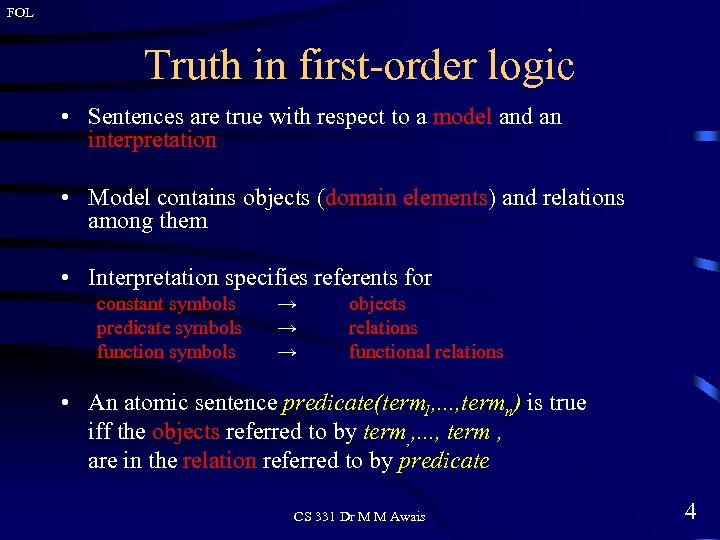FOL Truth in first-order logic • Sentences are true with respect to a model and an interpretation • Model contains objects (domain elements) and relations among them • Interpretation specifies referents for constant symbols predicate symbols function symbols → → → objects relations functional relations • An atomic sentence predicate(term 1, . . . , termn) is true iff the objects referred to by term, , . . . , term , are in the relation referred to by predicate CS 331 Dr M M Awais 4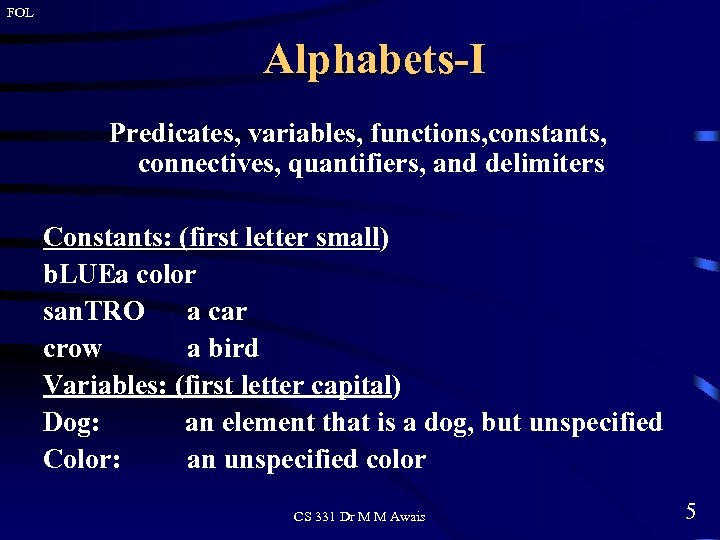FOL Alphabets-I Predicates, variables, functions, constants, connectives, quantifiers, and delimiters Constants: (first letter small) b. LUEa color san. TRO a car crow a bird Variables: (first letter capital) Dog: an element that is a dog, but unspecified Color: an unspecified color CS 331 Dr M M Awais 5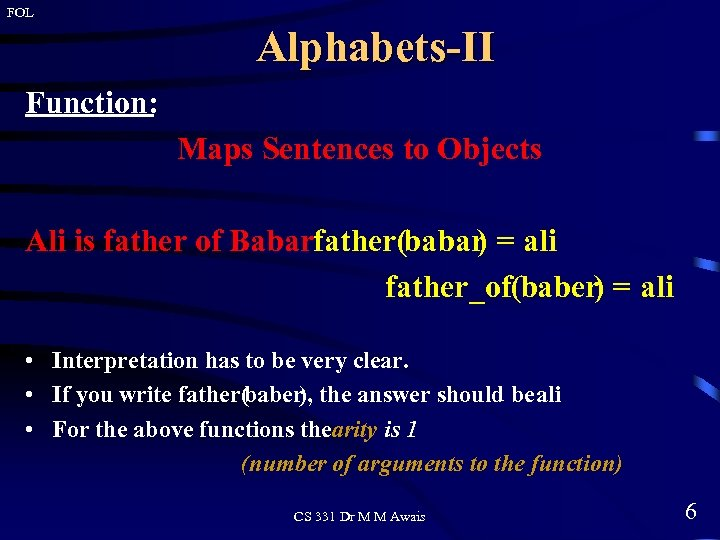FOL Alphabets-II Function: Maps Sentences to Objects Ali is father of Babarfather(babar = ali ) father_of(baber) = ali • Interpretation has to be very clear. • If you write father( baber), the answer should be ali • For the above functions thearity is 1 (number of arguments to the function) CS 331 Dr M M Awais 6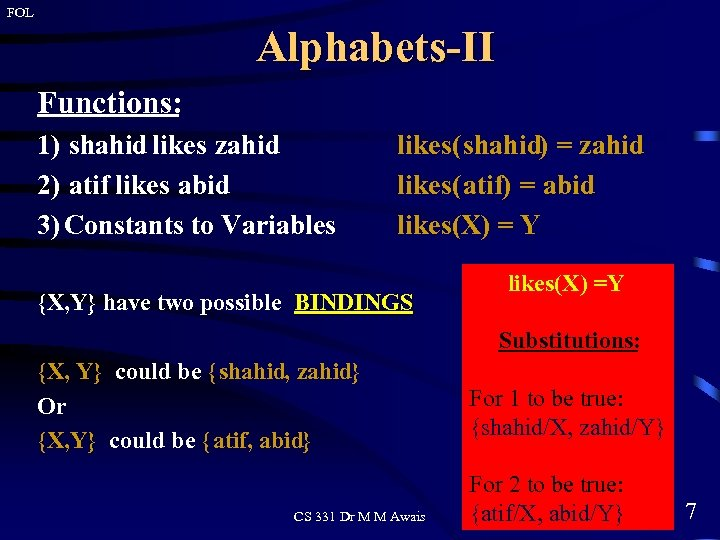FOL Alphabets-II Functions: 1) shahid likes zahid 2) atif likes abid 3) Constants to Variables likes(shahid) = zahid likes(atif) = abid likes(X) = Y {X, Y} have two possible BINDINGS likes(X) =Y Substitutions: {X, Y} could be { shahid, zahid} Or {X, Y} could be { atif, abid} CS 331 Dr M M Awais For 1 to be true: {shahid/X, zahid/Y} For 2 to be true: {atif/X, abid/Y} 7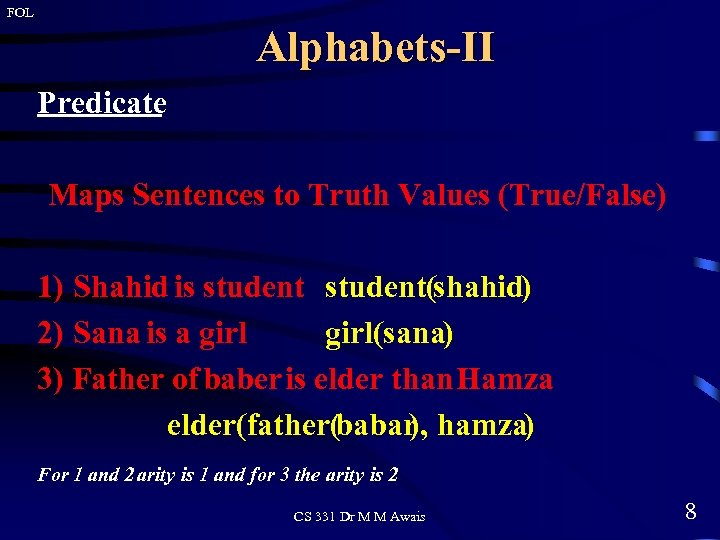FOL Alphabets-II Predicate Maps Sentences to Truth Values (True/False) 1) Shahid is student(shahid) 2) Sana is a girl(sana) 3) Father of baber is elder than Hamza elder(father(babar hamza) ), For 1 and 2 arity is 1 and for 3 the arity is 2 CS 331 Dr M M Awais 8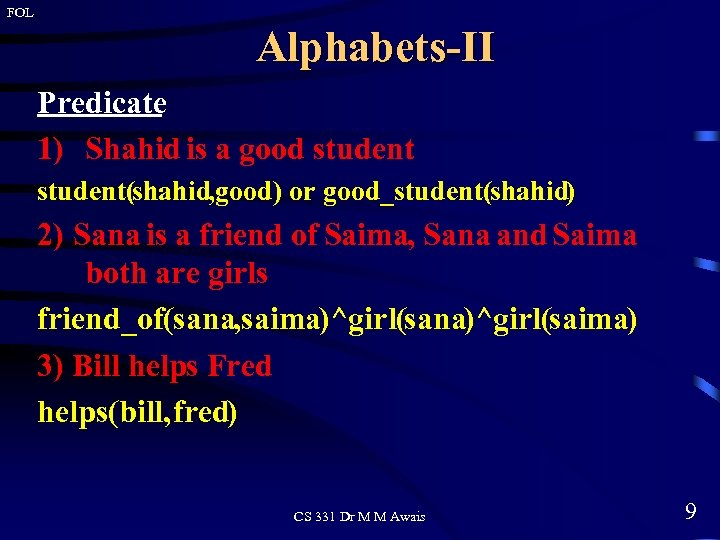FOL Alphabets-II Predicate 1) Shahid is a good student(shahid, good) or good_student(shahid) 2) Sana is a friend of Saima, Sana and Saima both are girls friend_of(sana, saima)^girl(sana)^girl(saima) 3) Bill helps Fred helps(bill, fred) CS 331 Dr M M Awais 9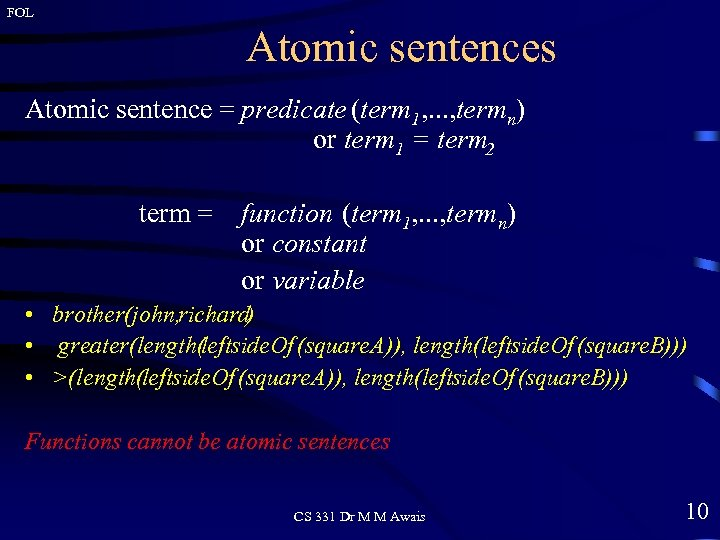FOL Atomic sentences Atomic sentence = predicate (term 1, . . . , termn) or term 1 = term 2 term = function (term 1, . . . , termn) or constant or variable • brother(john, richard) • greater(length(leftside. Of (square. A)), length(leftside. Of (square. B))) • >(length(leftside. Of (square. A)), length(leftside. Of (square. B))) Functions cannot be atomic sentences CS 331 Dr M M Awais 10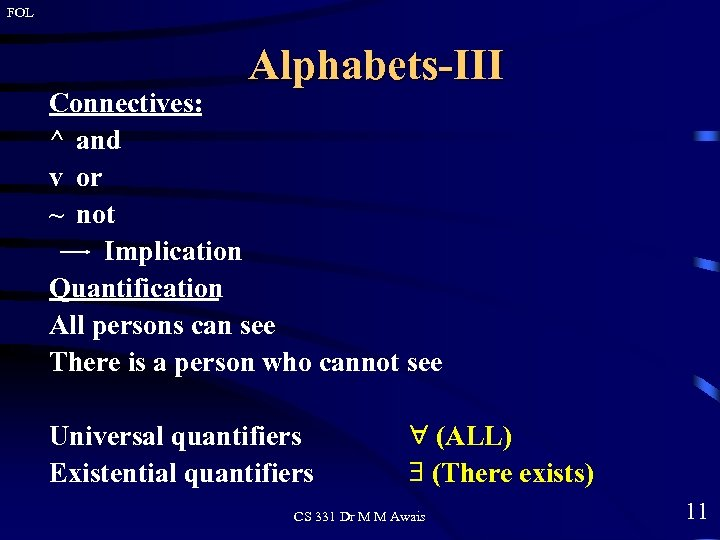FOL Alphabets-III Connectives: ^ and v or ~ not Implication Quantification All persons can see There is a person who cannot see Universal quantifiers Existential quantifiers (ALL) (There exists) CS 331 Dr M M Awais 11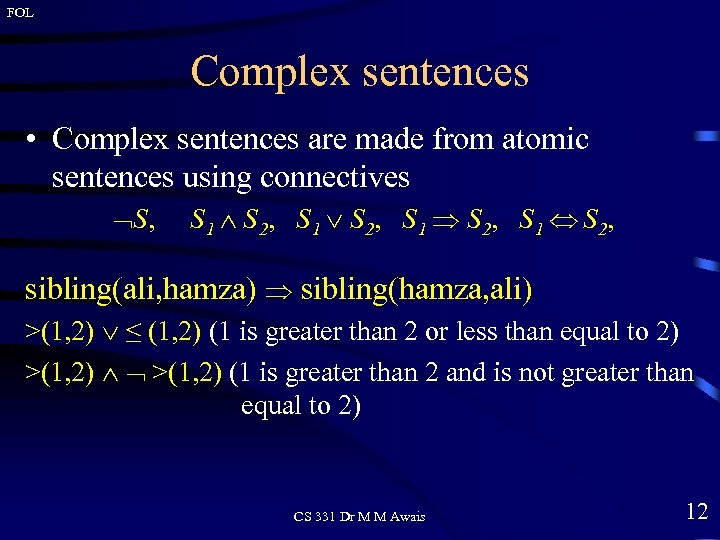FOL Complex sentences • Complex sentences are made from atomic sentences using connectives S, S 1 S 2, sibling(ali, hamza) sibling(hamza, ali) >(1, 2) ≤ (1, 2) (1 is greater than 2 or less than equal to 2) >(1, 2) (1 is greater than 2 and is not greater than equal to 2) CS 331 Dr M M Awais 12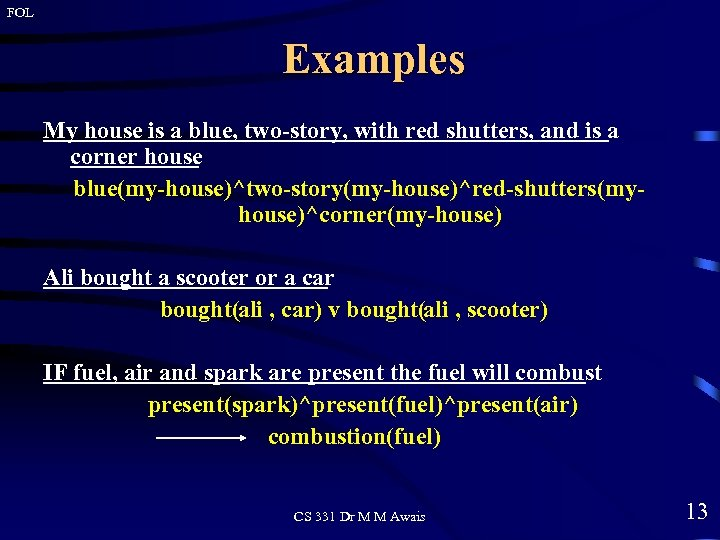FOL Examples My house is a blue, two-story, with red shutters, and is a corner house blue(my-house)^two-story(my-house)^red-shutters(myhouse)^corner(my-house) Ali bought a scooter or a car bought(ali , car) v bought(ali , scooter) IF fuel, air and spark are present the fuel will combust present(spark)^present(fuel)^present(air) combustion(fuel) CS 331 Dr M M Awais 13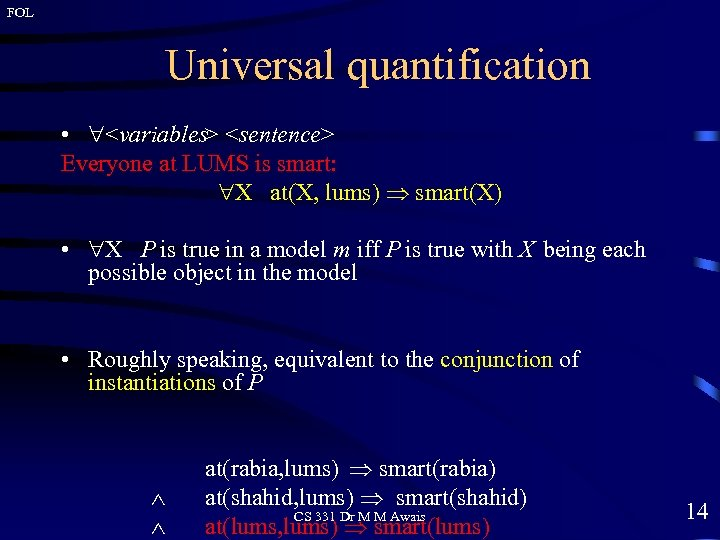FOL Universal quantification • Everyone at LUMS is smart: X at(X, lums) smart(X) • X P is true in a model m iff P is true with X being each possible object in the model • Roughly speaking, equivalent to the conjunction of instantiations of P at(rabia, lums) smart(rabia) at(shahid, lums) smart(shahid) CS 331 Dr M M Awais at(lums, lums) smart(lums) 14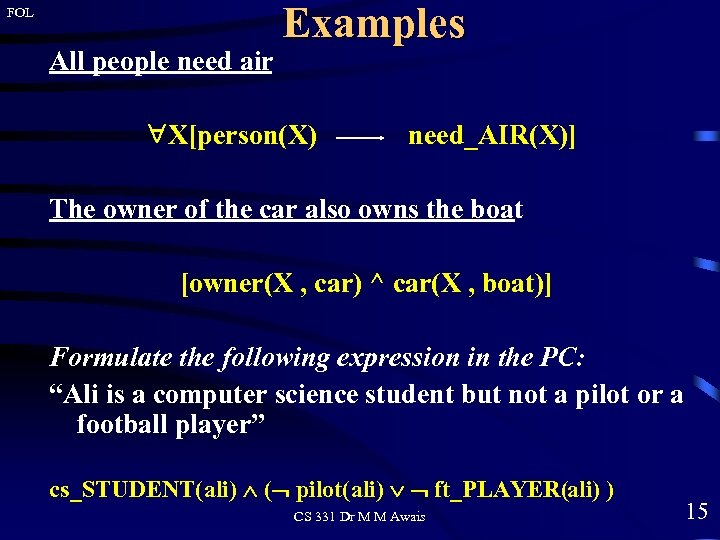FOL All people need air Examples X[person(X) need_AIR(X)] The owner of the car also owns the boat [owner(X , car) ^ car(X , boat)] Formulate the following expression in the PC: “Ali is a computer science student but not a pilot or a football player” cs_STUDENT(ali) ( pilot(ali) ft_PLAYER(ali) ) CS 331 Dr M M Awais 15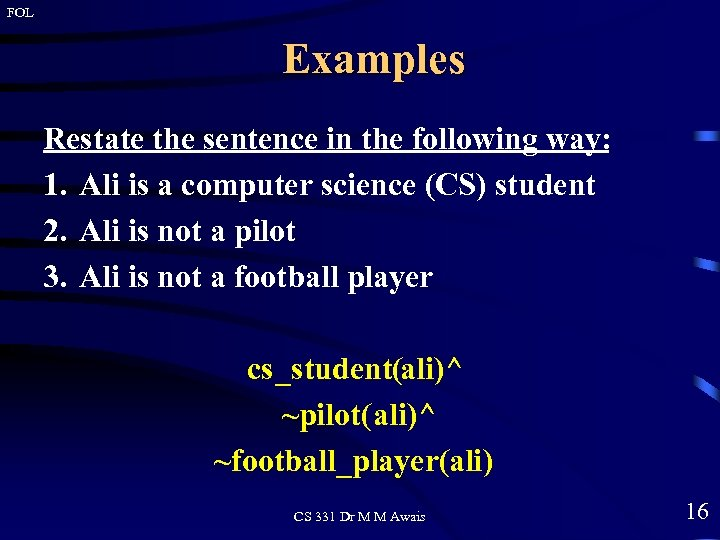FOL Examples Restate the sentence in the following way: 1. Ali is a computer science (CS) student 2. Ali is not a pilot 3. Ali is not a football player cs_student(ali)^ ~pilot(ali)^ ~football_player(ali) CS 331 Dr M M Awais 16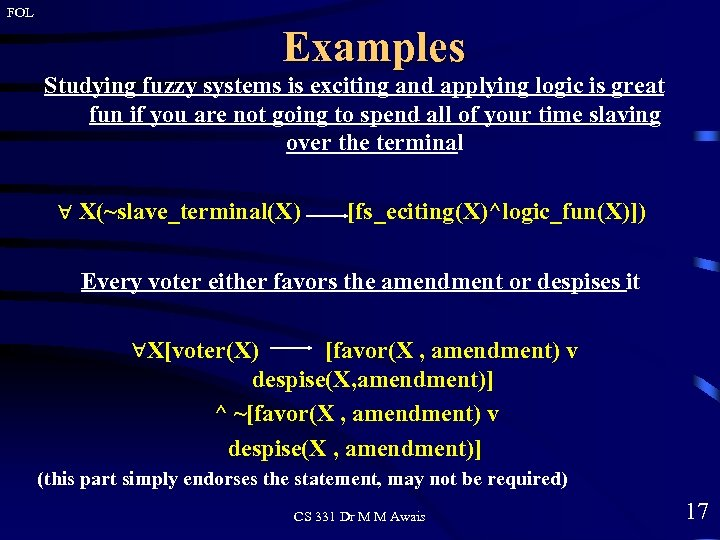FOL Examples Studying fuzzy systems is exciting and applying logic is great fun if you are not going to spend all of your time slaving over the terminal X(~slave_terminal(X) [fs_eciting(X)^logic_fun(X)]) Every voter either favors the amendment or despises it X[voter(X) [favor(X , amendment) v despise(X, amendment)] ^ ~[favor(X , amendment) v despise(X , amendment)] (this part simply endorses the statement, may not be required) CS 331 Dr M M Awais 17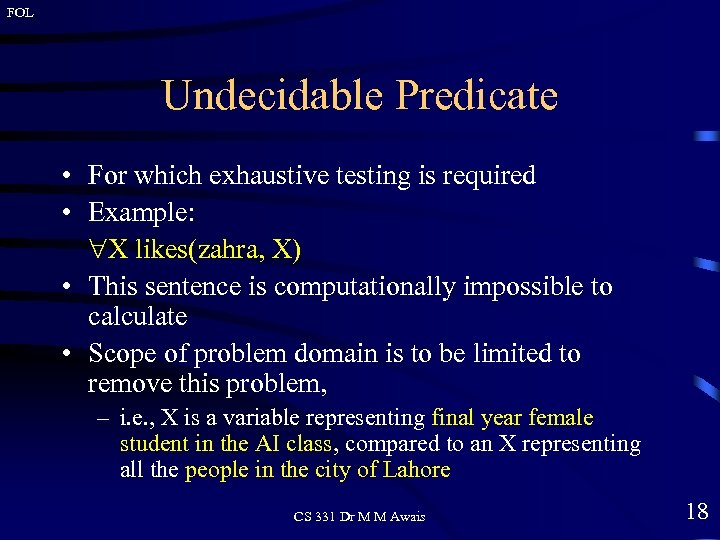FOL Undecidable Predicate • For which exhaustive testing is required • Example: X likes(zahra, X) • This sentence is computationally impossible to calculate • Scope of problem domain is to be limited to remove this problem, – i. e. , X is a variable representing final year female student in the AI class, compared to an X representing all the people in the city of Lahore CS 331 Dr M M Awais 18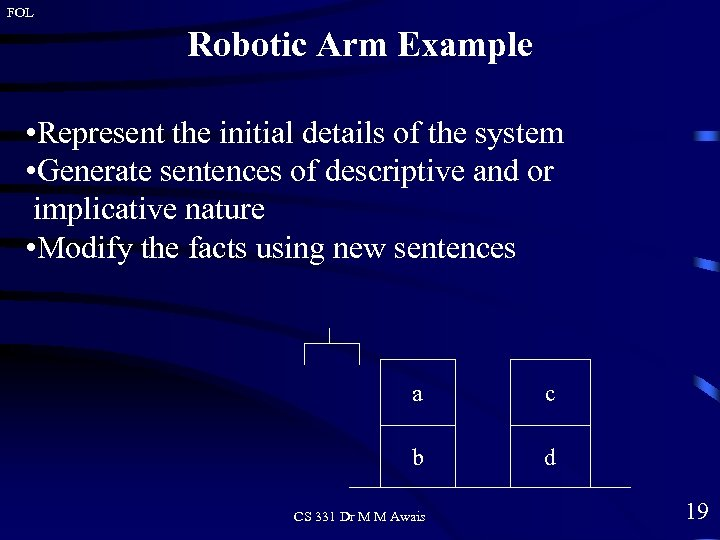FOL Robotic Arm Example • Represent the initial details of the system • Generate sentences of descriptive and or implicative nature • Modify the facts using new sentences a c b d CS 331 Dr M M Awais 19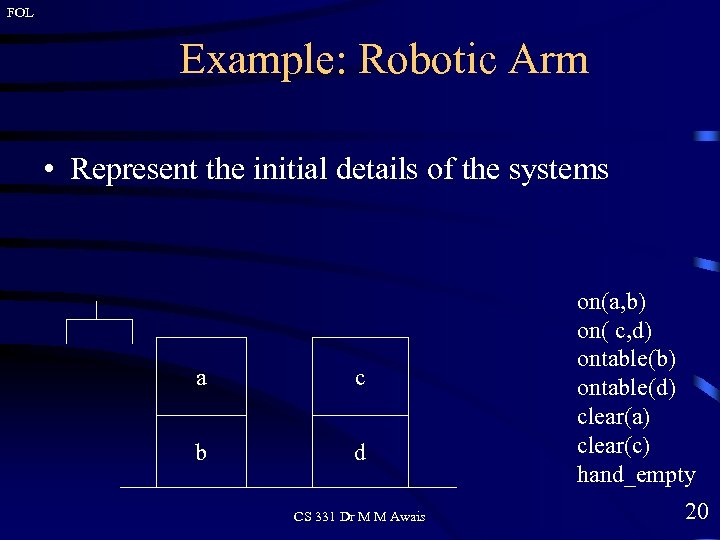FOL Example: Robotic Arm • Represent the initial details of the systems a c b d CS 331 Dr M M Awais on(a, b) on( c, d) ontable(b) ontable(d) clear(a) clear(c) hand_empty 20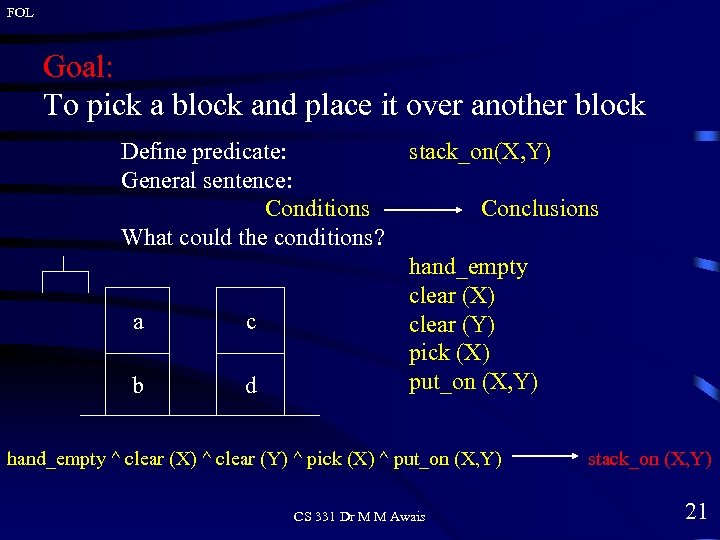FOL Goal: To pick a block and place it over another block Define predicate: General sentence: Conditions What could the conditions? a c b d stack_on(X, Y) Conclusions hand_empty clear (X) clear (Y) pick (X) put_on (X, Y) hand_empty ^ clear (X) ^ clear (Y) ^ pick (X) ^ put_on (X, Y) CS 331 Dr M M Awais stack_on (X, Y) 21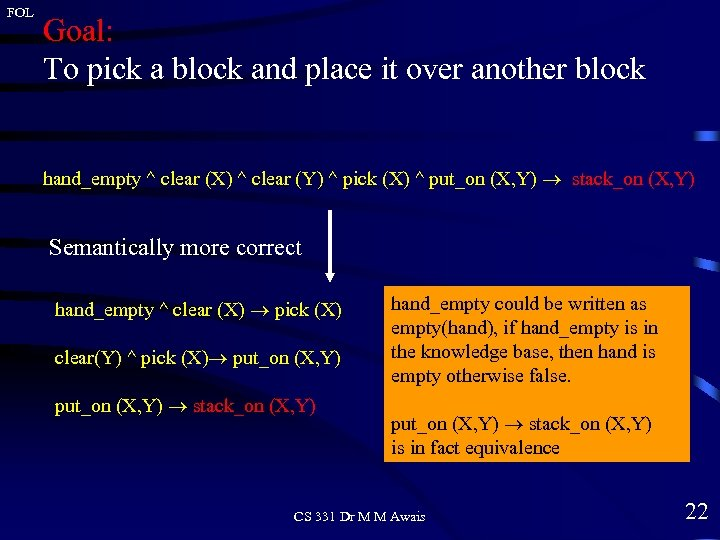FOL Goal: To pick a block and place it over another block hand_empty ^ clear (X) ^ clear (Y) ^ pick (X) ^ put_on (X, Y) stack_on (X, Y) Semantically more correct hand_empty ^ clear (X) pick (X) clear(Y) ^ pick (X) put_on (X, Y) stack_on (X, Y) hand_empty could be written as empty(hand), if hand_empty is in the knowledge base, then hand is empty otherwise false. put_on (X, Y) stack_on (X, Y) is in fact equivalence CS 331 Dr M M Awais 22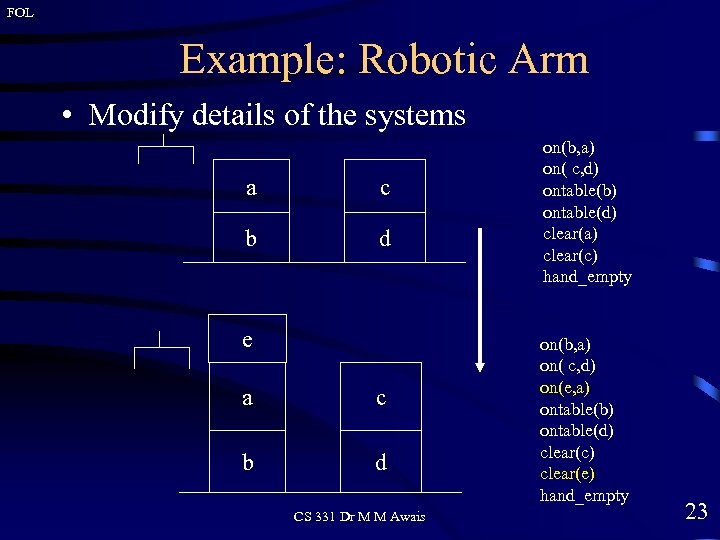FOL Example: Robotic Arm • Modify details of the systems a c b d e a c b d CS 331 Dr M M Awais on(b, a) on( c, d) ontable(b) ontable(d) clear(a) clear(c) hand_empty on(b, a) on( c, d) on(e, a) ontable(b) ontable(d) clear(c) clear(e) hand_empty 23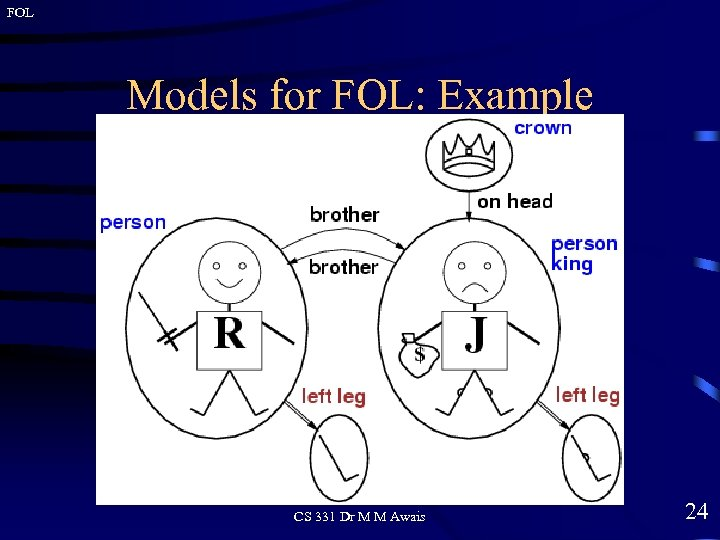FOL Models for FOL: Example CS 331 Dr M M Awais 24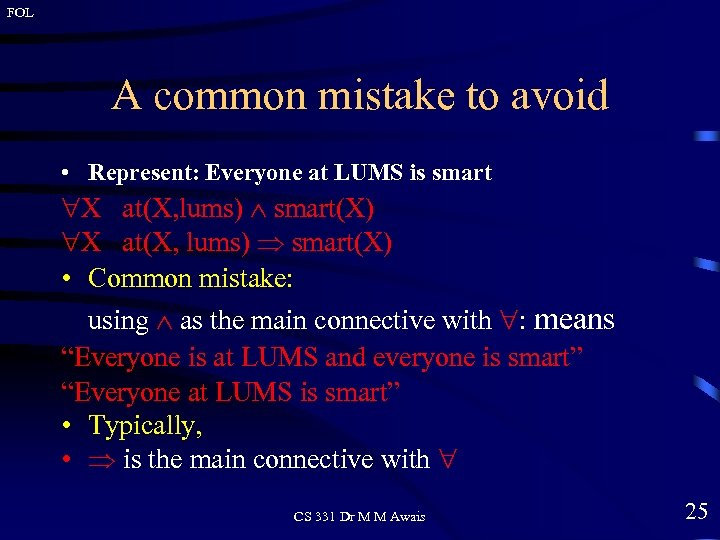FOL A common mistake to avoid • Represent: Everyone at LUMS is smart X at(X, lums) smart(X) • Common mistake: using as the main connective with : means “Everyone is at LUMS and everyone is smart” “Everyone at LUMS is smart” • Typically, • is the main connective with CS 331 Dr M M Awais 25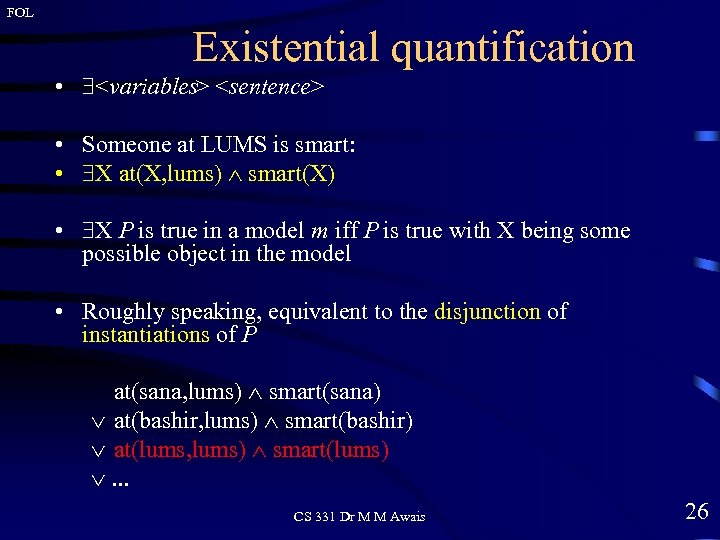FOL Existential quantification • • Someone at LUMS is smart: • X at(X, lums) smart(X) • X P is true in a model m iff P is true with X being some possible object in the model • Roughly speaking, equivalent to the disjunction of instantiations of P at(sana, lums) smart(sana) at(bashir, lums) smart(bashir) at(lums, lums) smart(lums) . . . CS 331 Dr M M Awais 26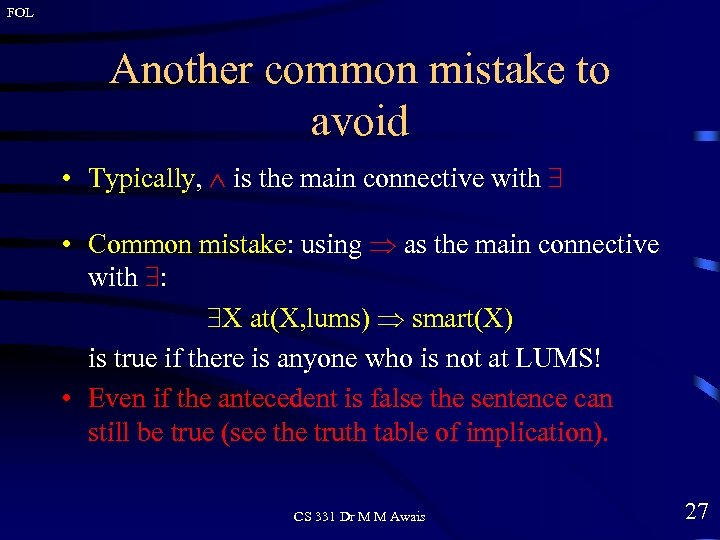FOL Another common mistake to avoid • Typically, is the main connective with • Common mistake: using as the main connective with : X at(X, lums) smart(X) is true if there is anyone who is not at LUMS! • Even if the antecedent is false the sentence can still be true (see the truth table of implication). CS 331 Dr M M Awais 27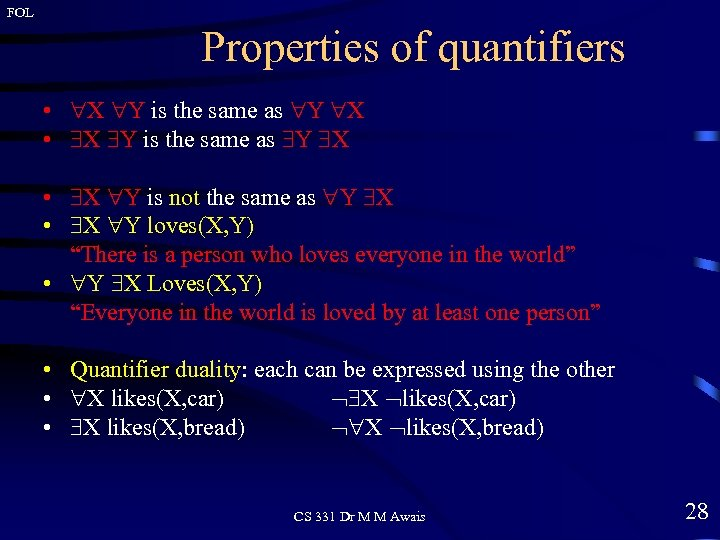FOL Properties of quantifiers • X Y is the same as Y X • X Y is not the same as Y X • X Y loves(X, Y) “There is a person who loves everyone in the world” • Y X Loves(X, Y) “Everyone in the world is loved by at least one person” • Quantifier duality: each can be expressed using the other • X likes(X, car) • X likes(X, bread) CS 331 Dr M M Awais 28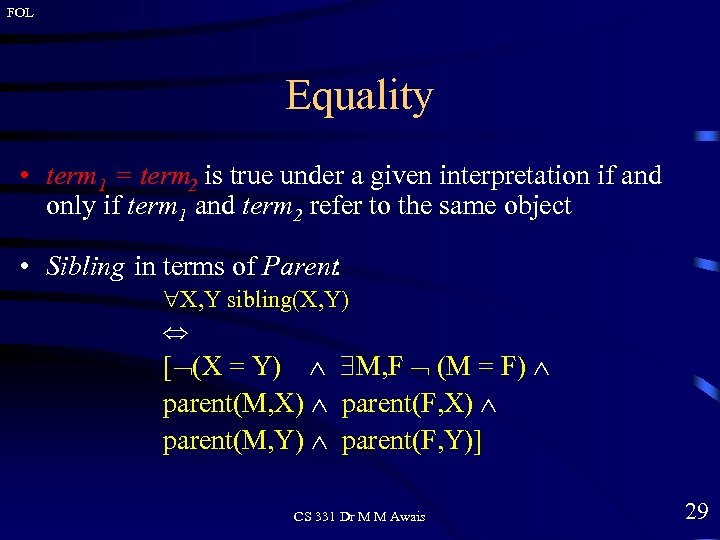FOL Equality • term 1 = term 2 is true under a given interpretation if and only if term 1 and term 2 refer to the same object • Sibling in terms of Parent: X, Y sibling(X, Y) [ (X = Y) M, F (M = F) parent(M, X) parent(F, X) parent(M, Y) parent(F, Y)] CS 331 Dr M M Awais 29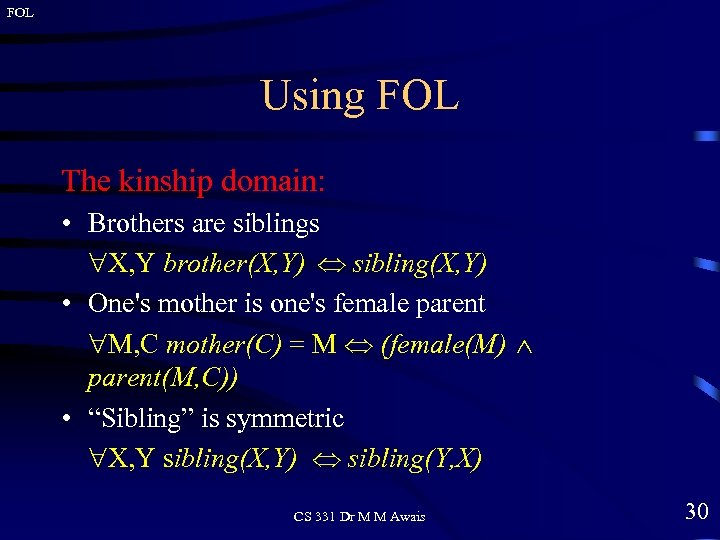FOL Using FOL The kinship domain: • Brothers are siblings X, Y brother(X, Y) sibling(X, Y) • One's mother is one's female parent M, C mother(C) = M (female(M) parent(M, C)) • “Sibling” is symmetric X, Y sibling(X, Y) sibling(Y, X) CS 331 Dr M M Awais 30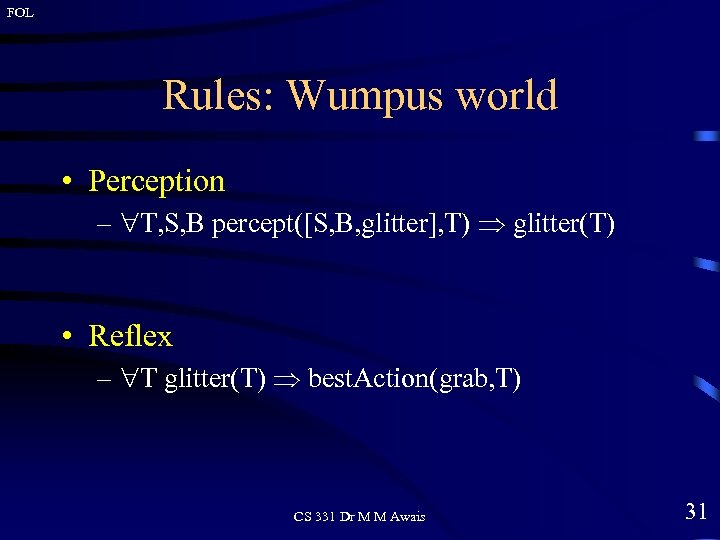FOL Rules: Wumpus world • Perception – T, S, B percept([S, B, glitter], T) glitter(T) • Reflex – T glitter(T) best. Action(grab, T) CS 331 Dr M M Awais 31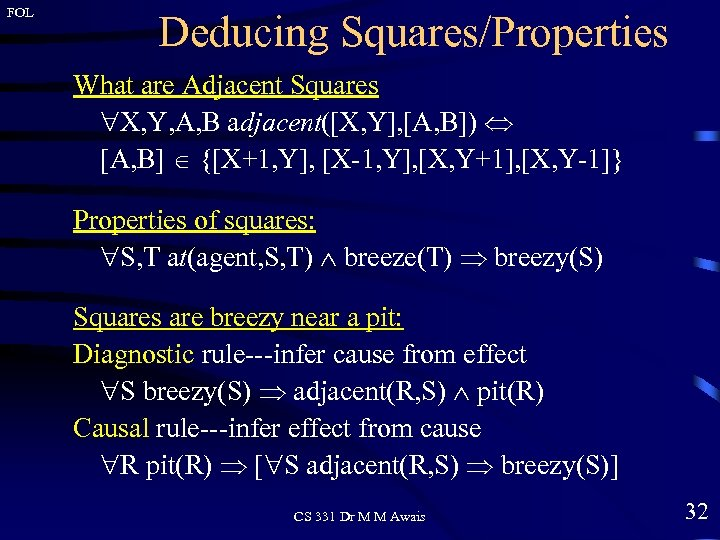FOL Deducing Squares/Properties What are Adjacent Squares X, Y, A, B adjacent([X, Y], [A, B]) [A, B] {[X+1, Y], [X-1, Y], [X, Y+1], [X, Y-1]} Properties of squares: S, T at(agent, S, T) breeze(T) breezy(S) Squares are breezy near a pit: Diagnostic rule---infer cause from effect S breezy(S) adjacent(R, S) pit(R) Causal rule---infer effect from cause R pit(R) [ S adjacent(R, S) breezy(S)] CS 331 Dr M M Awais 32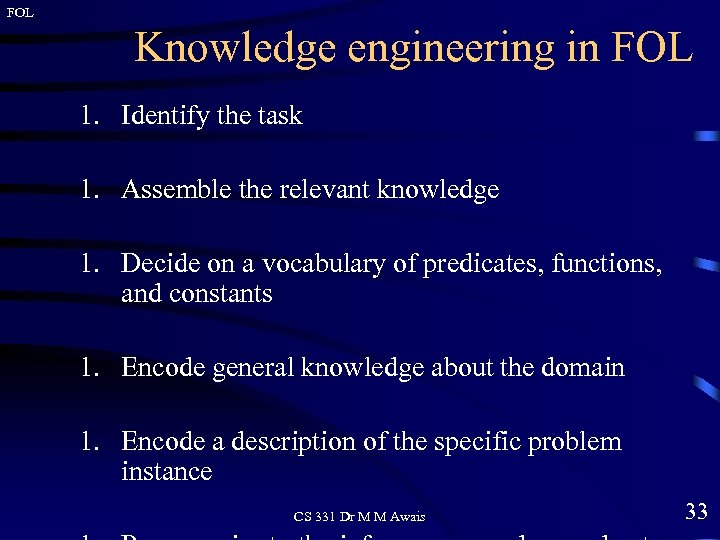FOL Knowledge engineering in FOL 1. Identify the task 1. Assemble the relevant knowledge 1. Decide on a vocabulary of predicates, functions, and constants 1. Encode general knowledge about the domain 1. Encode a description of the specific problem instance CS 331 Dr M M Awais 33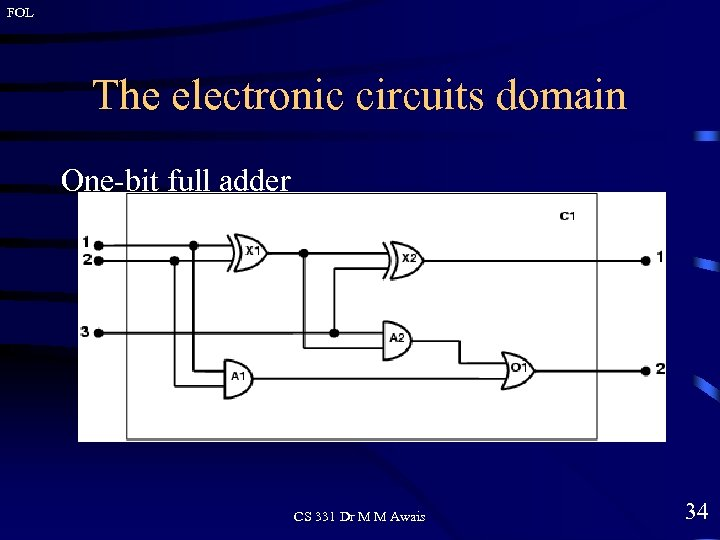FOL The electronic circuits domain One-bit full adder CS 331 Dr M M Awais 34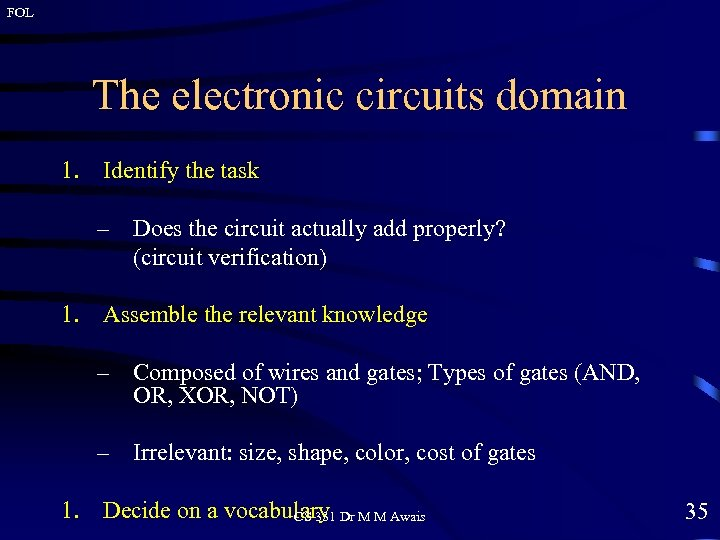FOL The electronic circuits domain 1. Identify the task – Does the circuit actually add properly? (circuit verification) 1. Assemble the relevant knowledge – Composed of wires and gates; Types of gates (AND, OR, XOR, NOT) – Irrelevant: size, shape, color, cost of gates 1. Decide on a vocabulary Dr M M Awais CS 331 35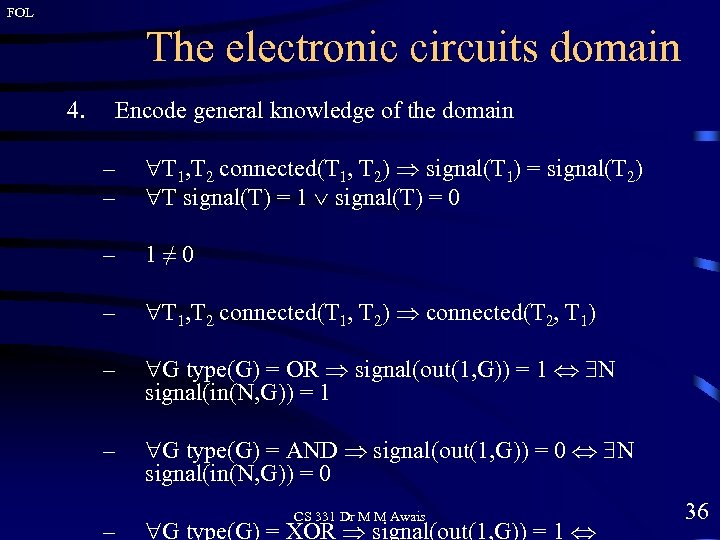FOL The electronic circuits domain 4. Encode general knowledge of the domain – – T 1, T 2 connected(T 1, T 2) signal(T 1) = signal(T 2) T signal(T) = 1 signal(T) = 0 – 1≠ 0 – T 1, T 2 connected(T 1, T 2) connected(T 2, T 1) – G type(G) = OR signal(out(1, G)) = 1 N signal(in(N, G)) = 1 – G type(G) = AND signal(out(1, G)) = 0 N signal(in(N, G)) = 0 – CS 331 Dr M M Awais G type(G) = XOR signal(out(1, G)) = 1 36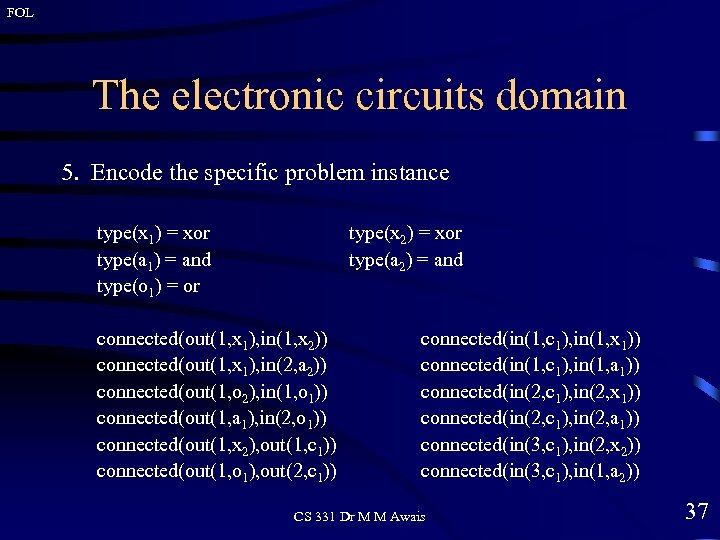FOL The electronic circuits domain 5. Encode the specific problem instance type(x 1) = xor type(a 1) = and type(o 1) = or type(x 2) = xor type(a 2) = and connected(out(1, x 1), in(1, x 2)) connected(out(1, x 1), in(2, a 2)) connected(out(1, o 2), in(1, o 1)) connected(out(1, a 1), in(2, o 1)) connected(out(1, x 2), out(1, c 1)) connected(out(1, o 1), out(2, c 1)) connected(in(1, c 1), in(1, x 1)) connected(in(1, c 1), in(1, a 1)) connected(in(2, c 1), in(2, x 1)) connected(in(2, c 1), in(2, a 1)) connected(in(3, c 1), in(2, x 2)) connected(in(3, c 1), in(1, a 2)) CS 331 Dr M M Awais 37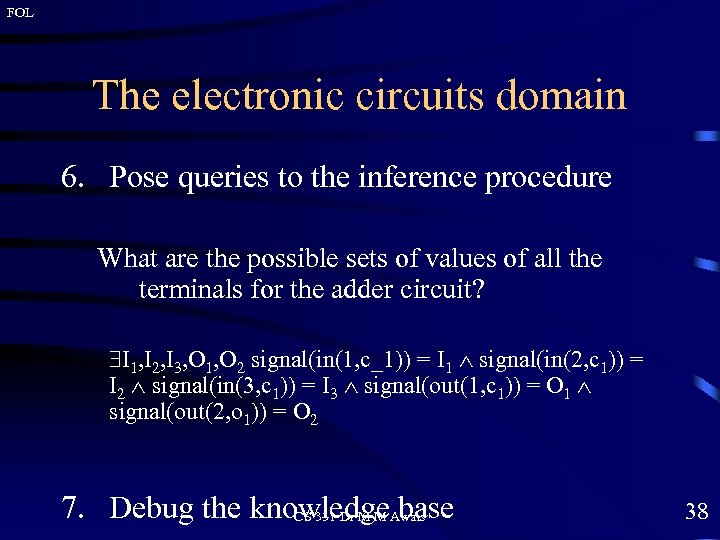FOL The electronic circuits domain 6. Pose queries to the inference procedure What are the possible sets of values of all the terminals for the adder circuit? I 1, I 2, I 3, O 1, O 2 signal(in(1, c_1)) = I 1 signal(in(2, c 1)) = I 2 signal(in(3, c 1)) = I 3 signal(out(1, c 1)) = O 1 signal(out(2, o 1)) = O 2 7. Debug the knowledge. Awais CS 331 Dr M M base 38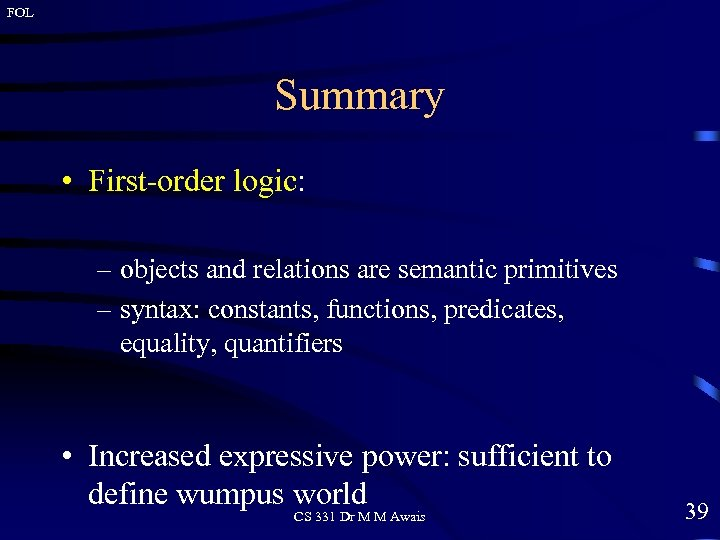FOL Summary • First-order logic: – objects and relations are semantic primitives – syntax: constants, functions, predicates, equality, quantifiers • Increased expressive power: sufficient to define wumpus world CS 331 Dr M M Awais 39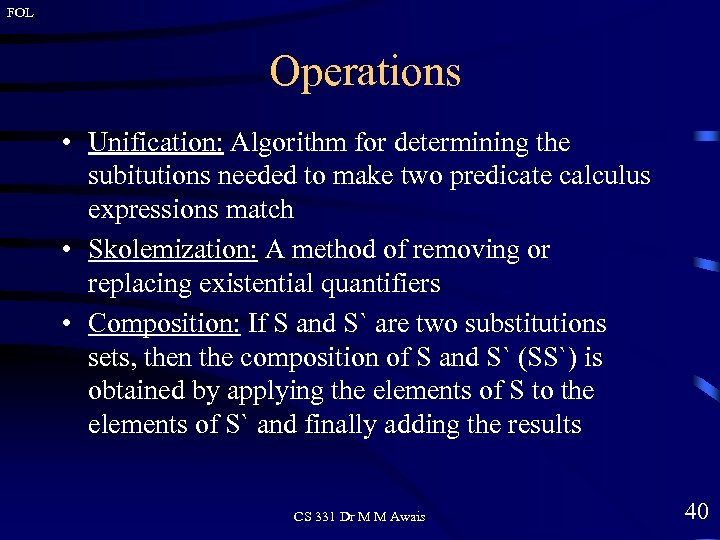FOL Operations • Unification: Algorithm for determining the subitutions needed to make two predicate calculus expressions match • Skolemization: A method of removing or replacing existential quantifiers • Composition: If S and S` are two substitutions sets, then the composition of S and S` (SS`) is obtained by applying the elements of S to the elements of S` and finally adding the results CS 331 Dr M M Awais 40